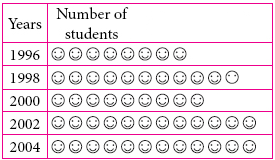Mathematics
Easy

Question

# Write the number of students enrolled in the year 2000.## 4769Hint:

## The correct answer is: 9

### Here we have to find the number of students enrolled in the year 2000.Firstly, In fig, we are given by two column . One is Years and another is Number of student,Look 2000 in Years column , It is on 3rd place so,Count the total number of students in same row of Years, Therefore, the total number of students enrolled in the year 2000 is 9.The correct answer is 9 (option (d)).In this question , We have given by table we just find out the number of students enrolled in the year 2000.

### Related Questions to study#### With Turito Foundation.#### Get an Expert Advice From Turito.